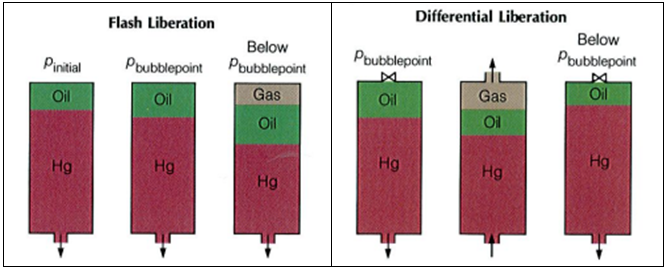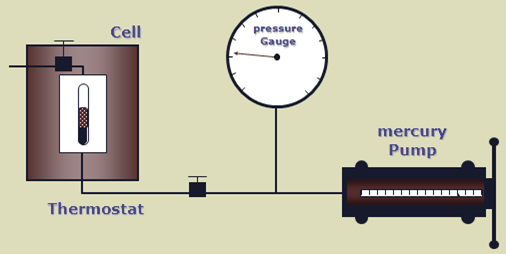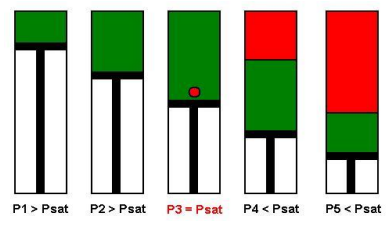## PVT Experiments: Differential Liberation

The bubble point pressure is determined by an experiment called “Constant Composition Expansion” (CCE), also called: “flash liberation”. The device used to perform this experiment is the PV cell, as shown and described in the article “Constant Composition Expansion “. The Differential Liberation (DL), discussed in this article, is experimentally performed in a similar PV cell.

The main difference between these two types of experiments is that in the Constant Composition Expansion (or flash expansion) no gas is removed from the PV cell. But instead, the gas remains in equilibrium with the oil. As a result, the overall hydrocarbon composition in the cell remains unchanged.

In the differential liberation experiment, however, pressure gradually decreases in steps and any liberated gas is removed from the oil. All depletion stages are performed at the same reservoir temperature. Therefore, there is a continual compositional change in the PV cell, the remaining hydrocarbons becoming progressively richer in the heavier components, and the average molecular weight thus increasing.The differential liberation experiment starts at the bubble point pressure determined from the CCE (since above this pressure the flash and differential experiments are identical).

### Example*:

The following example guides you on how to use and interpret the data from Differential Liberation test. The reservoir temperature is T= 200 °F and the bubble point pressure is 3330 psia.

The essential data obtained from the differential liberation experiment, performed on the same oil sample as CCE test, are listed in the following table:

## PVT Experiments: Constant Composition Expansion

Once we have a sample of reservoir fluid, either directly or by recombination, we need to do reservoir fluid measurement, often called PVT analysis. There are mainly 6 fluid properties measured: oil density, gas density, solution GOR, bubble point pressure, formation volume factor, and viscosity. This post will discuss how the bubble point pressure is measured and what the Constant Composition Expansion Experiment is?

### Experimental apparatus:

The bubble point pressure is determined by an experiment called Constant Composition Expansion (CCE). It’s also called Constant Mass Expansion (CME) experiment or Pressure-Volume (PV) relationship.

The apparatus used to perform this experiment is the PV cell, as shown in the figure below. The fluid is charged in the PV cell after recombining the oil and gas in the correct proportions. The temperature, controlled by a thermostat, is maintained constant throughout the experiment (the measured reservoir temperature). The cell pressure is controlled by a positive displacement pump and recorded on an accurate pressure gauge.### Bubble Point Pressure:

The bubble point pressure is the pressure, at some reference temperature, that the first bubble of gas is liberated from the liquid phase. It is also known as the saturation pressure.
The reference temperature is usually the reservoir temperature, but any temperature can be used.
Note that the bubble point pressure is a function of temperature and changing the reference temperature will change the bubble point pressure.

### Bubble Point Measurement:

A schematic of a Constant Composition Expansion experiment steps is shown in the figure below. The PV cell pressure is initially raised to a value greater than the bubble point pressure. the pressure is subsequently reduced in stages, and on each stage, the volume of the cell is recorded.